# Problems of the Week

Contribute a problem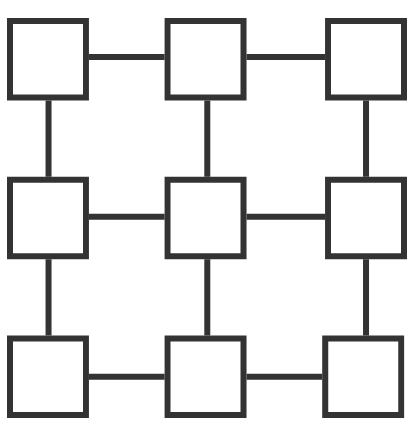Use distinct positive integers to fill in the grid such that any 2 connected squares have a greatest common divisor that is not 1.

What is the smallest possible value of the largest positive integer used?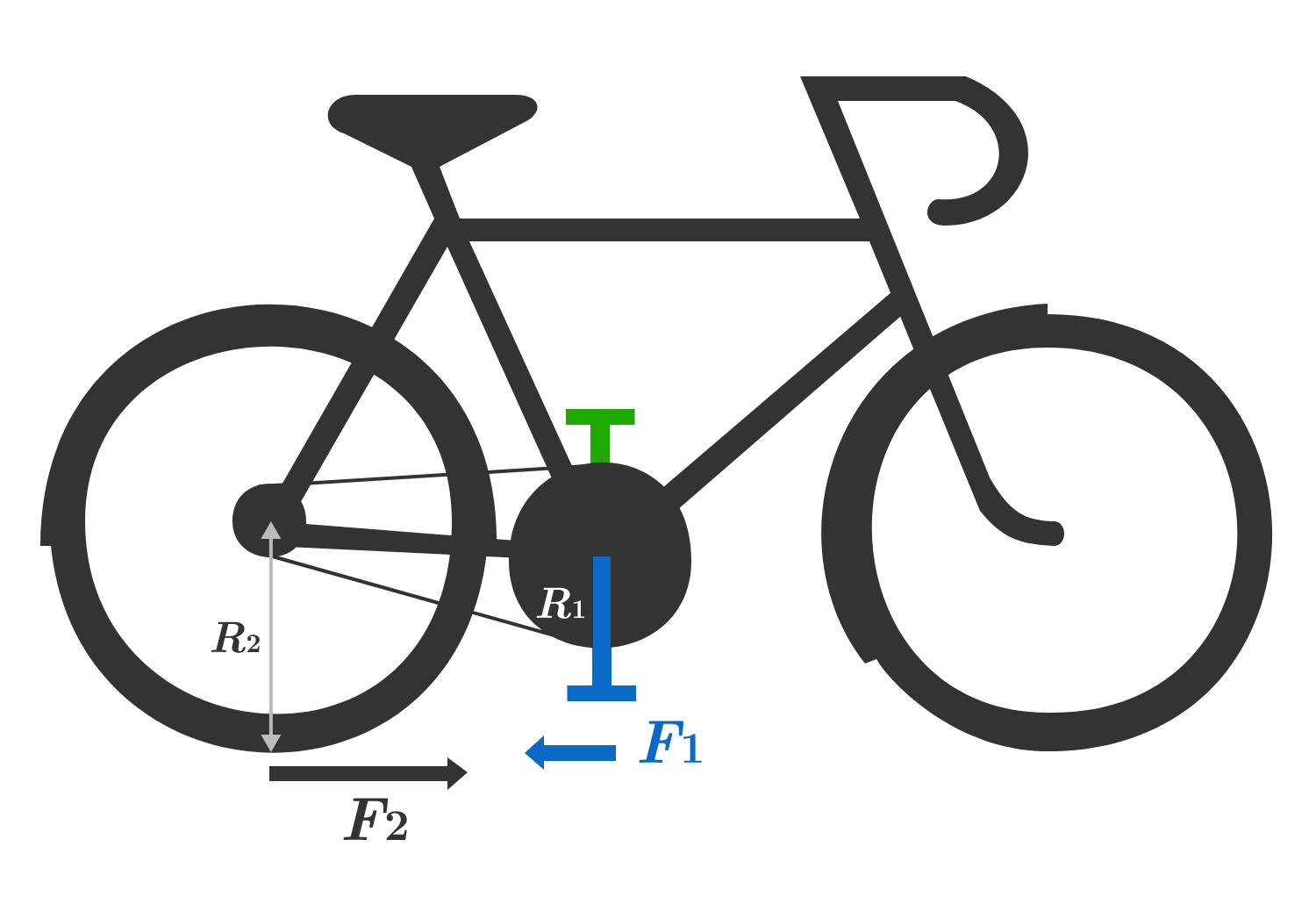A bicycle rests with its front tire on a frictionless surface and its rear tire on rough pavement, which provides friction $F_{2}$ so that the rear tire rolls without slipping.

An external force $F_{1}= \SI{100}{\newton}$ is applied to the blue pedal in the rearward direction at a distance of $R_{1}= \SI{0.10}{\meter}$ from the center of the sprocket, which has negligible moment of inertia.

Taking rotation to be positive in the counterclockwise direction, what is the instantaneous angular acceleration of the rear wheel, $\alpha,$ in $\si[per-mode=symbol]{\radian\per\second\squared}?$ Submit your answer to 1 decimal place.

Assumptions and Details

• The moment of inertia of the rear wheel is $I=\SI{0.50}{\kilogram \meter \squared}.$
• The wheel has a radius of $R_{2}=\SI{0.35}{\meter}$ which is also the height of the center of the sprocket above the ground.
• The mass of the bicycle is $M=\SI{15}{\kilogram}$.
• Assume there is no other friction in the system.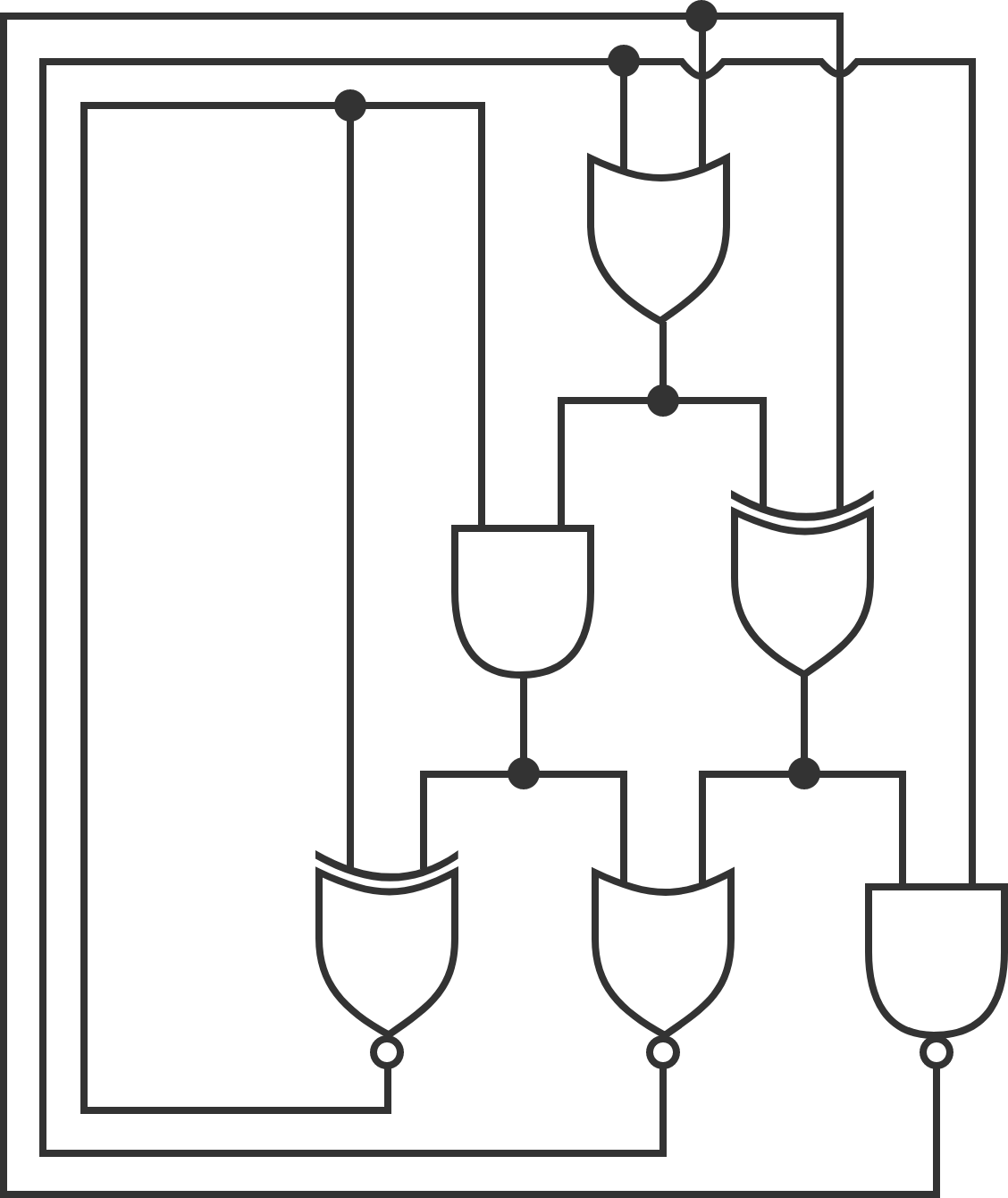The above is a logic circuit, made up of logic gates. Each wire holds either the value true or the value false. A black dot indicates that the wires meeting at that point have the same value. The six big things are logic gates; each receives two inputs from the top side and produces a value at the bottom. The gates can be seen in the above page; note that the three bottom gates have a white dot at the bottom (their outputs are inverted).

Only one configuration of the logic circuit shown is stable. Determine this configuration. Enter your answer as a 6-digit binary number representing the output of each gate (starting from the single gate on the top row, then the two on the middle row from left to right, then the three on the bottom row from left to right), where 1 = true and 0 = false. (Leading zeros are ok.)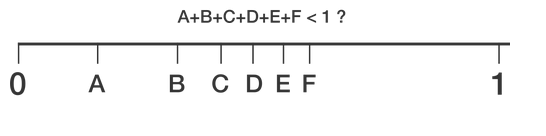Six variables $x_i$ are chosen uniformly at random in the interval $[0,1]$.

Find the probability that $x_1 + x_2 + x_3 + x_4 + x_5 + x_6 < 1.$ If the answer is of the form $\frac{a}{b}$, where $a$ and $b$ are coprime positive integers, what is $a+b?$

###### Inspired by Brian Charlesworth

The Triangle Cookie Company in the historic district of the Old Town has been making its perfectly round cookies for decades and selling them in their signature isosceles triangle boxes, with three rows separated by straight red packing strips, following these two packaging rules: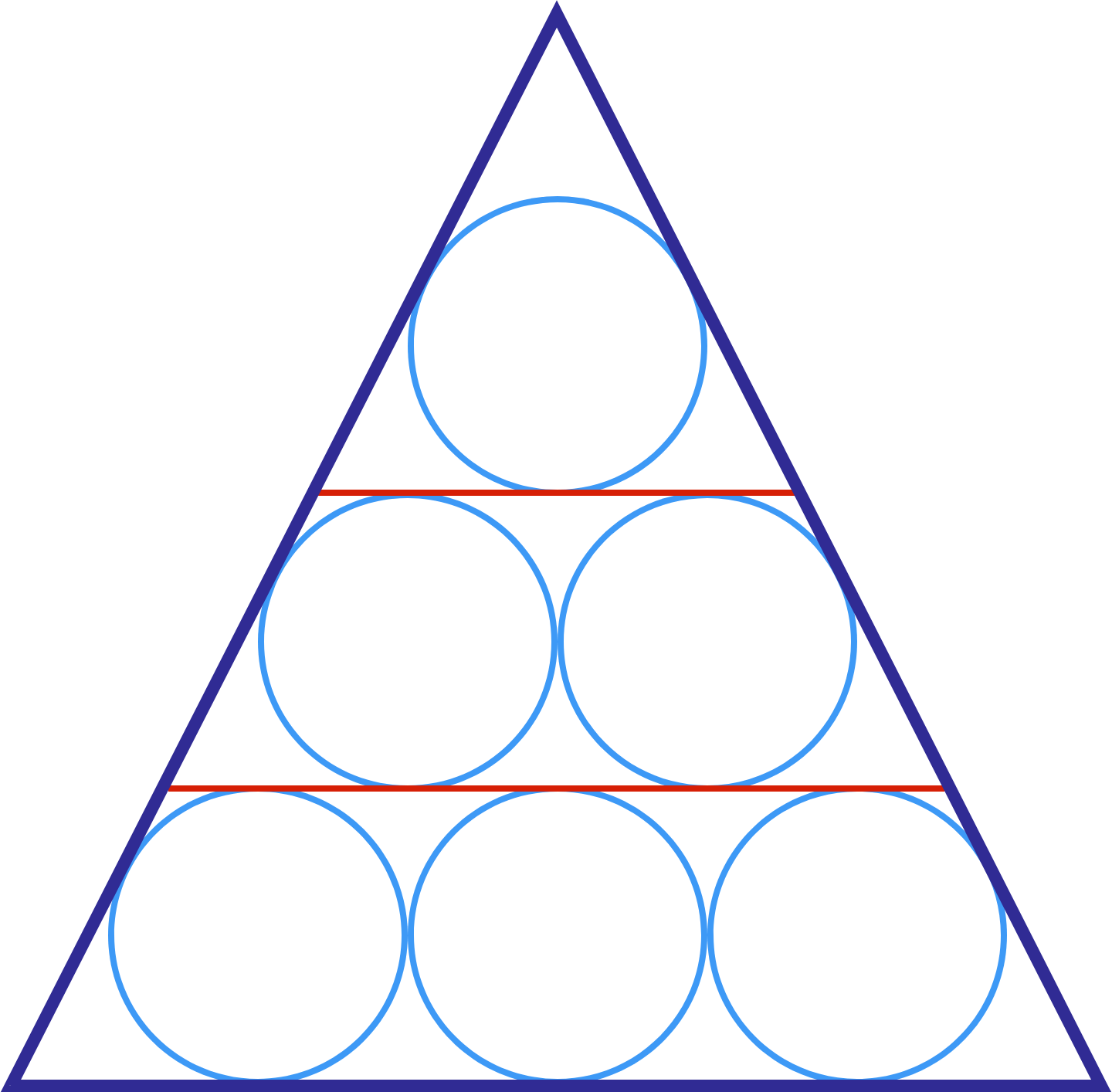1. All of the cookies are exactly the same size.
2. All of the cookies in the same row are tangent to each other, to the red strips, and to the sides of the boxes, with no gaps.

An enterprising employee decides to try improving the packing efficiency--measured by the ratio of the area occupied by the cookies to the area of the box--by relaxing the first rule to "All of the cookies in the same row are exactly the same size." This means that each row could have cookies of a different diameter than the other $2$ rows. The cookies will be packaged in an isosceles triangle box with suitable dimensions, so that it obeys the second rule.

Let $R$ be the packing efficiency. Then, approximately, what's the maximum possible percentage increase in $R$ obtained by adjusting the sizes of the round cookies instead of keeping them all the same?

×